# Neural Networks and Deep Learning（二）BP网络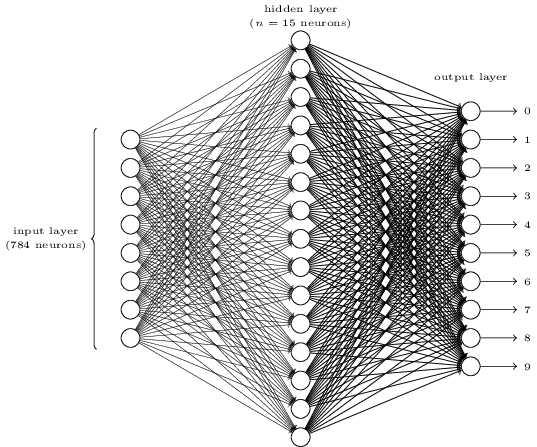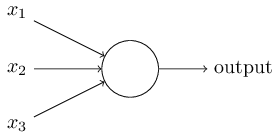比如上面的一个神经元，它的输出就是：

$\begin{eqnarray}\mbox{output} = \left\{ \begin{array}{ll}0 & \mbox{if} \sum_j w_j x_j \leq \mbox{ threshold} \\1 & \mbox{if} \sum_j w_j x_j > \mbox{threshold}\end{array}\right.\tag{1}\end{eqnarray}$

$\begin{eqnarray}\mbox{output} = \left\{ \begin{array}{ll} 0 & \mbox{if } w\cdot x + b \leq 0 \\1 & \mbox{if } w\cdot x + b > 0\end{array}\right.\tag{2}\end{eqnarray}$$\begin{eqnarray} \sigma(z) \equiv \frac{1}{1+e^{-z}}\tag{3}\end{eqnarray}$

sigmmoid函数还有一个优点就是它的导数很好计算，可以用它本身来表示：

$\begin{eqnarray}\sigma'(z)=\sigma(z)(1-\sigma(z))\tag{4}\end{eqnarray}$

BP网络的参数就是所有连线上的权重w和所有神经元中的激活阈值b，如果知道这些参数，给定一个输入x，则可以很容易的通过正向传播（feedforward）的方法计算到输出，即不断的执行$w \cdot x + b$操作，然后用sigmoid激活，再把上一层的输出传递给下一层作为输入，直到最后一层。

def feedforward(self, a):
"""Return the output of the network if a is input."""
for b, w in zip(self.biases, self.weights):
a = sigmoid(np.dot(w, a)+b)
return a


$\begin{eqnarray} C(w,b) \equiv\frac{1}{2n} \sum_x \| y(x) - a\|^2\tag{5}\end{eqnarray}$

$\begin{eqnarray}w_k & \rightarrow & w_k' = w_k-\eta \frac{\partial C}{\partial w_k} \tag{6}\\b_l & \rightarrow & b_l' = b_l-\eta \frac{\partial C}{\partial b_l}.\tag{7}\end{eqnarray}$

def __init__(self, sizes):
self.num_layers = len(sizes)
self.sizes = sizes
self.biases = [np.random.randn(y, 1) for y in sizes[1:]]
self.weights = [np.random.randn(y, x)
for x, y in zip(sizes[:-1], sizes[1:])]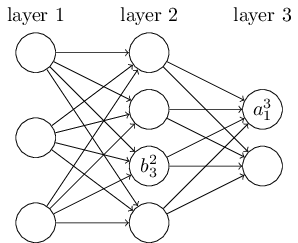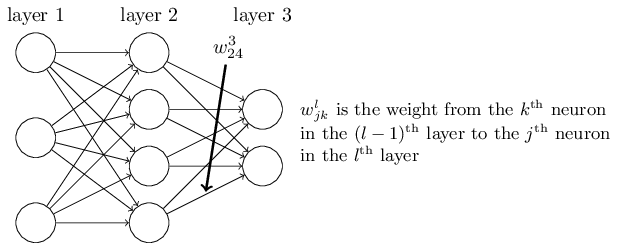$\begin{eqnarray} z^{l}_j = \sum_k w^{l}_{jk} a^{l-1}_k + b^l_j \tag{8}\end{eqnarray}$

$\begin{eqnarray} a^{l}_j =\sigma (z^{l}_j) = \sigma\left( \sum_k w^{l}_{jk} a^{l-1}_k + b^l_j \right)\tag{9}\end{eqnarray}$

$\begin{eqnarray} z^l=w^la^{l-1}+b^l \tag{10}\end{eqnarray}$

$\begin{eqnarray} a^{l} = \sigma (z^l) = \sigma\left( w^la^{l-1}+b^l \right)\tag{11}\end{eqnarray}$

$\begin{eqnarray}C = \frac{1}{2} \|y-a^L\|^2 = \frac{1}{2} \sum_j (y_j-a^L_j)^2.\tag{12}\end{eqnarray}$

$\begin{eqnarray} \delta^l_j \equiv \frac{\partial C}{\partial z^l_j}.\tag{13}\end{eqnarray}$

$\begin{eqnarray} \delta^L_j = \frac{\partial C}{\partial z^L_j} = \frac{\partial C}{\partial a^L_j}\frac{\partial a^L_j}{\partial z^L_j} =\frac{\partial C}{\partial a^L_j} \sigma'(z^L_j).\tag{14}\end{eqnarray}$

$\begin{eqnarray} \delta^L = \nabla_a C \odot \sigma'(z^L).\tag{BP1}\end{eqnarray}$

$\begin{eqnarray}\left[\begin{array}{c} 1 \\ 2 \end{array}\right] \odot \left[\begin{array}{c} 3 \\ 4\end{array} \right]= \left[ \begin{array}{c} 1 * 3 \\ 2 * 4 \end{array} \right]= \left[ \begin{array}{c} 3 \\ 8 \end{array} \right].\tag{15}\end{eqnarray}$

$\begin{eqnarray}\delta^l_j & = & \frac{\partial C}{\partial z^l_j} \tag{16}\\& = & \sum_k \frac{\partial C}{\partial z^{l+1}_k} \frac{\partial z^{l+1}_k}{\partial z^l_j} \tag{17}\\ & = & \sum_k \frac{\partial z^{l+1}_k}{\partial z^l_j} \delta^{l+1}_k,\tag{18}\end{eqnarray}$

$\begin{eqnarray}z^{l+1}_k = \sum_j w^{l+1}_{kj} a^l_j +b^{l+1}_k = \sum_j w^{l+1}_{kj} \sigma(z^l_j) +b^{l+1}_k.\tag{19}\end{eqnarray}$

$\begin{eqnarray}\frac{\partial z^{l+1}_k}{\partial z^l_j} = w^{l+1}_{kj} \sigma'(z^l_j).\tag{20}\end{eqnarray}$

$\begin{eqnarray}\delta^l_j = \sum_k w^{l+1}_{kj} \delta^{l+1}_k \sigma'(z^l_j).\tag{21}\end{eqnarray}$

$\begin{eqnarray} \delta^l = ((w^{l+1})^T \delta^{l+1}) \odot \sigma'(z^l),\tag{BP2}\end{eqnarray}$

$\begin{eqnarray}\frac{\partial C}{\partial b^l_j} = \frac{\partial C}{\partial z^l_j}\frac{\partial z^l_j}{\partial b^l_j} = \delta^l_j \tag{BP3}\end{eqnarray}$

$\begin{eqnarray}\frac{\partial C}{\partial w^l_{jk}} = \frac{\partial C}{\partial z^l_j}\frac{\partial z^l_j}{\partial w^l_{jk}} = a^{l-1}_k \delta^l_j.\tag{BP4}\end{eqnarray}$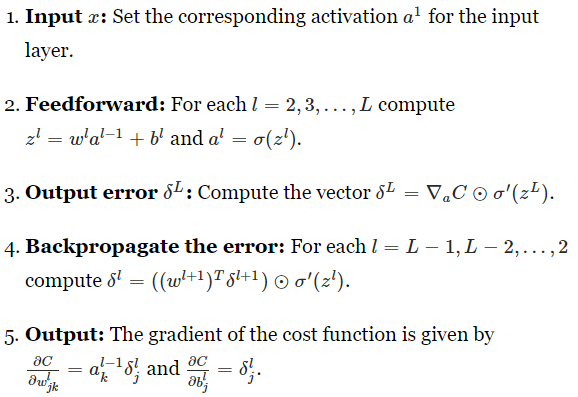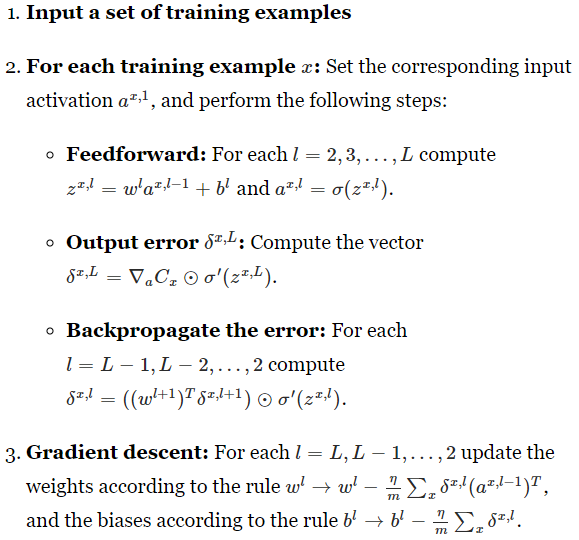$\begin{eqnarray} \delta^L = (a^L-y) \odot \{\sigma(z^L)(1-\sigma(z^L))\}.\tag{22}\end{eqnarray}$

net.SGD(training_data, 30, 10, 3.0, test_data=test_data)


## 8 thoughts on “Neural Networks and Deep Learning（二）BP网络”

1.anonymous

sigmoid函数已经不常用了，现在是relu或者LeakyRelu

1.admin Post author

是因为sigmoid的梯度消失吗？不过无论用什么激活函数，只要把(BP1)和(BP2)中的$\sigma(\cdot)$函数的导数换掉就好。

1.Anonymous

是的，首先是解决梯度消失问题，第二是能更快速收敛，而且不用复杂的计算。导数换掉是自然，只是这个领域发展太快，有的东西已经不再用了。

1.admin Post author

是的，领域发展太快了，所以作为入门的话，先从基础的、经典的学起比较合适。后续计划更新一些领域内的最新进展，欢迎交流。

This site uses Akismet to reduce spam. Learn how your comment data is processed.